# Just Enough Set Theory - Set Operations (Part 2 of 3)

Welcome to the second installment of this three-part series on set theory. The first piece, Set Theory Defined (recently updated with code samples), detailed requisite foundational knowledge. It is highly recommended that readers begin there if they haven’t already.

The first piece in this series introduced sets and exhibited how ES6 arrays are analogous to them. It also depicted how to transform, or map, a set into a related set. This post expands on set theory by probing into set operations.

NOTE: All code samples are written in ES6 and are therefore not likely to execute directly in a browser. The best option is to use Node or transpile the excerpts using either Babel or TypeScript. The working code is available on GitHub along with execution instructions.

## Empty Sets

Empty sets are a rather mundane topic, but nonetheless worth mentioning. As the name implies, they are simply sets that have no elements. They are also commonly referred to as null sets. Mathematically, empty sets are represented as either $\emptyset$ or $\{\}$. The concept relates to empty arrays in software.

## Cardinality

The term cardinality sounds impressive; however, it’s simply the number of elements in a set. The mathematical representation of a set with three elements is as depicted in Figure One – Cardinality.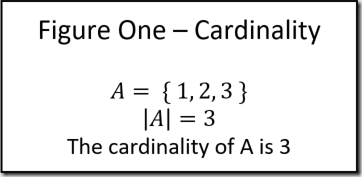In JavaScript, the cardinality of an array is its length. See the code below.

const someSet = [1, 2, 3, 4, 5];
const cardinality = someSet.length;
// cardinality = 5


## Subsets

Subsets are relatively easy to explain, yet have far reaching implications. A subset is a portion of a larger set. For instance, consider the set of all animals ($A$). The set of all dogs ($D$) is a subset of the animal set because although every animal is not a dog, every dog is an animal. The mathematical notation for subsets is as follows: $D \subseteq A$. Another way of mathematically expressing the subset relationship is $\forall x(x\in D \implies x\in A)$. That looks absurd, but the premise is that for any ($\forall$) element ($x$) in $D$, it is implied ($\implies$) that the element ($x$) also exists in $A$.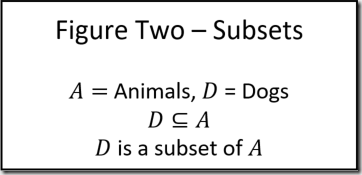Subsets are often taught with Venn Diagrams. See Figure Three – Venn Diagrams for an example. Admittedly, this account of subsets is a bit prosaic. However, the final post in this series relies heavily on the concept so it bears belaboring the point.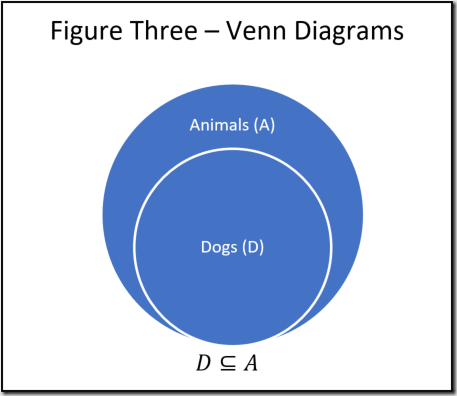ES6 has a built-in filter method on the array object that enables easy access to subsets. Filter takes a predicate as an argument. Recall from the first article that a predicate is a function that takes a single argument and returns a Boolean response. The filter method applies the predicate to each item in a set and creates a new set that includes the items where the predicate returned true. See the code below.

const animals = [
{name: "Tom", type: "Cat"},
{name: "Jerry", type: "Mouse"},
{name: "Pluto", type: "Dog"},
{name: "Scooby Doo", type: "Dog"}];

const dogs = animals.filter(a => a.type == "Dog");
// dogs = [{name: "Pluto", type: "Dog"}, {name: "Scooby Doo", type: "Dog"}]


## Summation

The term summation is a bit misleading because it implies simply adding elements together, however it’s a more powerful concept. Summation applies a function to each element of a set reducing it to a single value. $\sum_{x \in S}f(x)$ is the mathematical notation representing the algorithm where $S$ can be any set and $f(x)$ can be any function. Consider Figure Four – Summation. Given the set $A$ , each element in the set is multiplied by two and added together.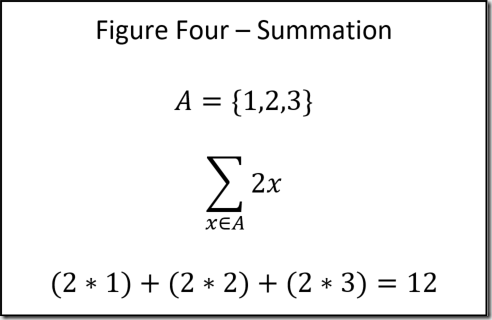ES6’s reduce method of the array object is comparable to summation. Aptly named, reduce applies a function to each member of a set reducing it to a single value. It accepts two arguments: a function and an optional starting value. The function accepts an accumulated value and the current item. The state of the accumulated value after all items are processed is the final return value. The code below is the same process detailed in Figure Four – Summation.

const someSet = [1, 2, 3];
const sum = someSet.reduce((acc, x) => acc + x * 2, 0);
// sum = 12


Reduce is useful for many operations beyond mathematical functions. The code below utilizes it to extract email addresses from a set of users.

const users = [
{id: 1, email: "email@email.com"},
{id: 2, email: "email2@email2.com"},
{id: 3, email: "email3@email.com"}];

const emails = users.map(u => u.email).reduce((acc, x) => ${acc};${x});
// emails = "email@email.com;email2@email2.com;email3@email.com"


This above doesn’t do the reduce method proper justice because its efficacy is virtually endless. There are many more options that are outside the scope of this feature. The reader is highly encouraged to find more information on Mozilla’s excellent JavaScript reference.

## Power Set

Power sets are something every programmer has to deal with at some point in his/her career, even if they can’t formally identify them by name. In mathematical parlance, power sets are denoted as $P(A)$. A power set is the set of all subsets including the empty set and itself: more succinctly, all possible set combinations. A power set always contains $2^n$ elements where $n$ is the cardinality of the original set ($|P(A)|=2^{|A|}$).

Power sets are difficult to conceptualize without an example. Figure Five – Power Set depicts a set with three elements. The power set is all possible combinations of the three elements. The result is a set with a cardinality of eight ($2^3$).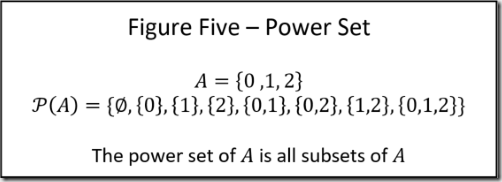Unfortunately, there isn’t an innate JavaScript method for creating power sets. However, that’s an easy problem to overcome given some ingenuity. See the code below.

const someSet = [0, 1, 2];
const powerSet = someSet.reduce((acc, x) => [...acc, ...acc.map(y => [x, ...y])], [[]]);
// powerSet = [[], , , [1,0], , [2,0], [2,1], [2,1,0]]


The code above is a bit intimidating at first glance so it merits additional explanation. The power set always contains an empty set, so the second argument to the reduce method is a set that contains nothing but that. This is the starting value. When the function acts on the first item in the set, the value of acc is [[]] and the value of x is 0. The result of concatenating the current item to each item in acc is concatenated on to the value of acc making it [[], ]. The same algorithm is applied to each item in the set. This is difficult to envisage, so the code below details essentially what happens upon invocation.

const ps = (acc, x) => [...acc, ...acc.map(y => [x, ...y])];

// First element
let acc = ps([[]], 0);
// acc = [[], ]

// Second element
acc = ps(acc, 1);
// acc = [[], , , [1,0]]

// Third element
acc = ps(acc, 2);
// acc = [[], , , [1, 0], , [2, 0], [2, 1], [2, 1, 0]]


## Conclusion

The post outlined a few useful set operations. ES6 uses the reduce method to apply the concept of summation to sets. A power set is a set of all possible set combinations. Although there is no built in ES6 functionality for this, it’s an easy algorithm to create. Make sure to come back for the final post entitled When Sets Collide. It is by far the most useful in the series covering set operations that act on multiple individual sets.